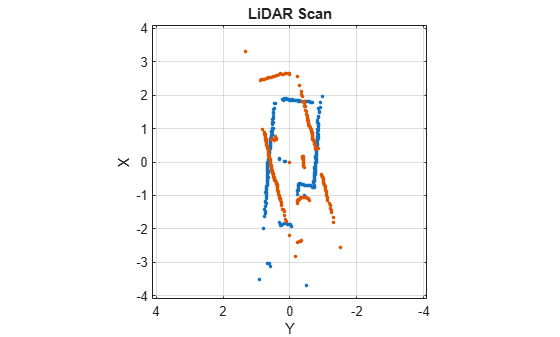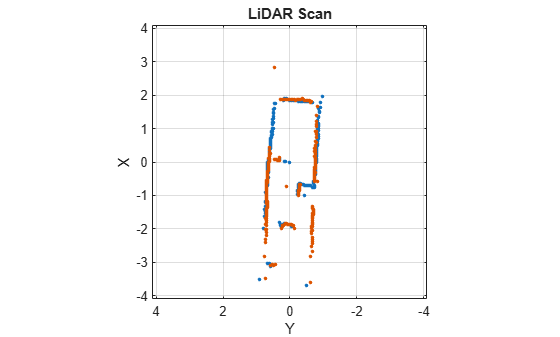# transformScan

Transform laser scan based on relative pose

## Syntax

``transScan = transformScan(scan,relPose)``
``````[transRanges,transAngles] = transformScan(ranges,angles,relPose)``````

## Description

example

````transScan = transformScan(scan,relPose)` transforms the laser scan specified in `scan` by using the specified relative pose, `relPose`.```

example

``````[transRanges,transAngles] = transformScan(ranges,angles,relPose)``` transforms the laser scan specified in `ranges` and `angles` by using the specified relative pose, `relPose`.```

## Examples

collapse all

Create a `lidarScan` object. Specify the ranges and angles as vectors.

```refRanges = 5*ones(1,300); refAngles = linspace(-pi/2,pi/2,300); refScan = lidarScan(refRanges,refAngles);```

Translate the laser scan by an `[x y]` offset of `(0.5,0.2)`.

`transformedScan = transformScan(refScan,[0.5 0.2 0]);`

Rotate the laser scan by 20 degrees.

`rotateScan = transformScan(refScan,[0,0,deg2rad(20)]);`

Use the `matchScans` function to find the relative transformation between two lidar scans. Then, transform the second scan into the coordinate frame of the first scan.

Load a pair of lidar scans as a pair of `lidarScan` objects. They are two scans of the same scene with a change in relative pose.

```load tb3_scanPair.mat plot(s1) hold on plot(s2) hold off```The relative pose is estimated from an odometry sensor and provided as a variable, `initGuess`, as `[x y theta]`.

`disp(initGuess)`
``` -0.7000 0.1500 -0.3254 ```

Use scan matching to find the relative pose between the two laser scans and specify the initial guess for the pose.

```pose = matchScans(s2,s1,"InitialPose",initGuess); disp(pose)```
``` -0.7213 0.1160 -0.2854 ```

Transform the second scan to the coordinate frame of the first scan. Plot the two scans to see that they now overlap.

```s2Transformed = transformScan(s2,pose); plot(s1) hold on plot(s2Transformed) hold off```## Input Arguments

collapse all

Lidar scan readings, specified as a `lidarScan` object.

Range values from scan data, specified as a vector in meters. These range values are distances from a sensor at specified `angles`. The vector must be the same length as the corresponding `angles` vector.

Angle values from scan data, specified as a vector in radians. These angle values are the specific angles of the specified `ranges`. The vector must be the same length as the corresponding `ranges` vector.

Relative pose of current scan, specified as ```[x y theta]```, where `[x y]` is the translation in meters and `theta` is the rotation in radians.

## Output Arguments

collapse all

Transformed lidar scan readings, specified as a `lidarScan` object.

Range values of transformed scan, returned as a vector in meters. These range values are distances from a sensor at specified `transAngles`. The vector is the same length as the corresponding `transAngles` vector.

Angle values of transformed scan, returned as a vector in radians. These angle values are the specific angles of the specified `transRanges`. The vector is the same length as the corresponding `ranges` vector.

## Version History

Introduced in R2017a